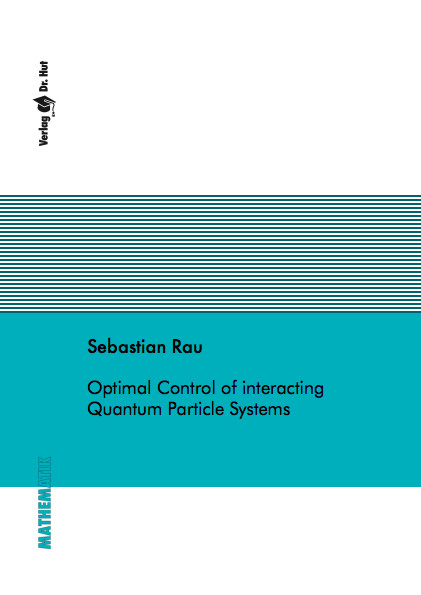Datenbestand vom 20. August 2019aktualisiert am 20. August 2019

# ISBN 9783843911504Euro 60,00 inkl. 7% MwSt

978-3-8439-1150-4, Reihe Mathematik

Sebastian Rau
Optimal Control of interacting Quantum Particle Systems

123 Seiten, Dissertation Technische Universität Kaiserslautern (2013), Softcover, A5

## Zusammenfassung / Abstract

This thesis deals with optimal control problems (OCP) for interacting quantum particle systems. We consider miscroscopic and macroscopic models, which are self-consistently coupled to the Poisson equation for the electrostatic potential. In Molecular Biology, one is interested in controlling a system of quantum particles to enforce the activation or breaking of chemical bonds. We therefore study a transient nonlinear Schr\"odinger-Poisson system (NLSP). Existence and uniqueness of solutions to the state system are shown. Formulating an optimal control problem we show the existence of a minimizer and the well-posedness of first-order optimality conditions. Numerical schemes exploiting the adjoint-based derivative are presented and supplemented with numerical examples. Turning to a macroscopic approach we consider the transient quantum drift-diffusion model (TQDD) and formally derive the first-order optimality conditions, followed by numerical examples. On the other hand, we consider the optimal design question of semiconductor devices by discussing the stationary quantum Euler-Poisson (QEP) model. Using available results for the existence of solutions to the state system we show the solvability of an optimization problem and of the linearized and adjoint states with the Fredholm alternative. First-order optimality conditions are given and used to numerically examine an example for a MESFET semiconductor device. Finally, we study optimal control problems constrained by the zero-relaxation time limit of the QEP, i.e. the stationary quantum drift-diffusion model. We perform the semiclassical limit, that is the transition from quantum to classical models, and show that minimizers and minima for QDD constrained OCPs converge to minimizers and minima constrained by the DD model. For all presented optimization algorithms we discuss the independence on the chosen discretization and the effect of the regularization.